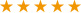Closed

Prompt：

1、When you download the e-book for the first time, the browser will prompt ‘Do you want to launch the external application’, click ‘Yes’, and remember my choice.

2、When the reader program is installed, some antivirus software will prompt ‘Link Browser’, select ‘Allow’and continue.

Confirm
------Collected by------

UCLA

University of Cambridge

Yale University

University of Melbourne Library

University of Oxford

Columbia University Library

National Library of China

Stanford University

UCB

Numerical Methods for Engineers, Second Edition

Griffiths, D. Vaughan

Classification Codes : TB

Language : ENG

Publication Information : CRC Press

Publish Year：2006

I  S  B  N :9781584884019

Grade :Contents
Product Details

Although pseudocodes, Mathematica®, and MATLAB® illustrate how algorithms work, designers of engineering systems write the vast majority of large computer programs in the Fortran language. Using Fortran 95 to solve a range of practical engineering problems, Numerical Methods for Engineers, Second Edition provides an introduction to numerical methods, incorporating theory with concrete computing exercises and programmed examples of the techniques presented.

Covering a wide range of numerical applications that have immediate relevancy for engineers, the book describes forty-nine programs in Fortran 95. Many of the programs discussed use a sub-program library called nm_lib that holds twenty-three subroutines and functions. In addition, there is a precision module that controls the precision of calculations.

Well-respected in their field, the authors discuss a variety of numerical topics related to engineering. Some of the chapter features include…

• The numerical solution of sets of linear algebraic equations
• Roots of single nonlinear equations and sets of nonlinear equations
• Numerical quadrature, or numerical evaluation of integrals
• An introduction to the solution of partial differential equations using finite difference and finite element approaches

Describing concise programs that are constructed using sub-programs wherever possible, this book presents many different contexts of numerical analysis, forming an excellent introduction to more comprehensive subroutine libraries such as the numerical algorithm group (NAG).